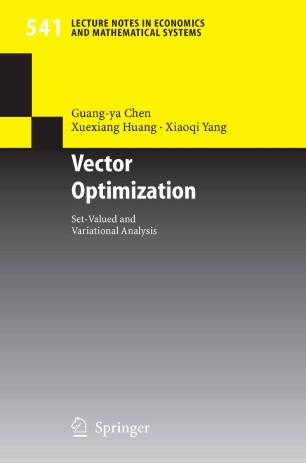# Vector Optimization

## Set-Valued and Variational Analysis

• Guang-ya Chen
• Xuexiang Huang
• Xiaoqi YangBook

Part of the Lecture Notes in Economics and Mathematical Systems book series (LNE, volume 541)

1. Front Matter
Pages I-X
2. Pages 37-110
3. Pages 111-182
4. Pages 183-254
5. Pages 255-270
6. Pages 271-290
7. Back Matter
Pages 291-308

### Introduction

Vector optimization model has found many important applications in decision making problems such as those in economics theory, management science, and engineering design (since the introduction of the Pareto optimal solu­ tion in 1896). Typical examples of vector optimization model include maxi­ mization/minimization of the objective pairs (time, cost), (benefit, cost), and (mean, variance) etc. Many practical equilibrium problems can be formulated as variational in­ equality problems, rather than optimization problems, unless further assump­ tions are imposed. The vector variational inequality was introduced by Gi- nessi (1980). Extensive research on its relations with vector optimization, the existence of a solution and duality theory has been pursued. The fundamental idea of the Ekeland's variational principle is to assign an optimization problem a slightly perturbed one having a unique solution which is at the same time an approximate solution of the original problem. This principle has been an important tool for nonlinear analysis and optimization theory. Along with the development of vector optimization and set-valued optimization, the vector variational principle introduced by Nemeth (1980) has been an interesting topic in the last decade. Fan Ky's minimax theorems and minimax inequalities for real-valued func­ tions have played a key role in optimization theory, game theory and math­ ematical economics. An extension was proposed to vector payoffs was intro­ duced by Blackwell (1955).

### Keywords

Scalarization Method Vector Equilibrium Problem Vector Optimization Vector Variational Inequality Vector Variational Principle analysis operations research optimization

#### Authors and affiliations

• Guang-ya Chen
• 1
• Xuexiang Huang
• 2
• Xiaoqi Yang
• 3
1. 1.Institute of Systems ScienceChinese Academy of SciencesBeijingChina
2. 2.Department of Mathematics and Computer SciencesChongqing Normal UniversityChongqingChina
3. 3.Department of Applied MathematicsThe Hong Kong Polytechnic UniversityKowloon, Hong Kong